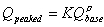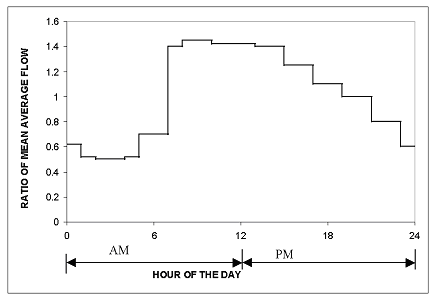#INFOSEWER

Manhole loads in InfoSewer and H2OMap Sewer

Manhole loads in InfoSewer and H2OMap Sewer

Wastewater loads represent the average flows that are applied to the sewer collection network from tributary areas. These loads are defined as the amount of wastewater generated from tributary areas that must be handled by the conveyance system. They are based on a knowledge of the area land use patterns, wastewater generation characteristics, industries, infiltration characteristics, external flows, etc. The wastewater loads are the basic data required for any hydraulic computation.

Wet-weather flow is related to rainfall activity and consists of groundwater infiltration (extraneous flow entering the sewer system because of poor construction, corrosion of the pipe, ground movement or structural failure through joints, porous walls or breaks) and structure inflow (extraneous flow entering the sewer system through manhole covers, basement drains and other sources).

For steady-state modeling, manhole loads can be either unpeaked or peaked as follows:

Unpeakable Flow Type - The corresponding load for each manhole is modeled as a direct flow into the sewer system.(15)

where Qbase represents the average base flow (in flow units).

Peakable Base Flow - H2OMAP Sewer uses a general form of the Federov's formula as follows:(16)

where K and r are peaking factor parameters. Default values are K = 2.4 and r = 0.89. Values of K and r can be modified.

Peakable Coverage Flow - H2OMAP Sewer uses the following formula which can describe both the Harman and Babbitt equations:(17)

where P represents the population and a, b, c, d and e are peaking parameters. The default values for these parameters are: a = 5; b = 0; c = 0.2, d = 0 and e = 1 which represents Babbitt equation1. For Harman equation1: a = 14; b = 4; c = 0.5, d = 1, and e = 1.

For an extended period simulation, no peaking formula is used, instead, the multiplication factors from the diurnal pattern are used to adjust (multiply) all types of loads before they are aggregated. An example peaking-factor curve is shown below.Infiltration and inflow affect the operation of a sanitary sewer system and pumping, treatment, and overflow regulators facilities.Infiltration occurs in gravity pipes while inflow occurs at manholes and wet wells. Infiltration loads refer to the volume of groundwater entering the sewer system from the soil through defective joints, broken or cracked pipes, improper connections, or manhole walls. Accurately determining infiltration is generally difficult as these loads depend on soil type, soil moisture conditions, system size and integrity, water table level, and the number of illegal connections. They are normally computed by subtracting base flow from total metered flow during dry weather or by compiling flow isolation measurements. Infiltration can be defined as proportional to the pipe length; proportional to the pipe length and to the pipe diameter; proportional to the pipe surface area (pipe length multiplied by its perimeter); proportional to the number of defects in the pipe (count-based); or as a pattern load/hydrograph (flow vs. time).

Inflow loads refer to stormwater or other drainage water and wastes (extraneous water) entering the sewer system through manhole covers. Inflow is measured during wet weather conditions and is determined by subtracting base flow and infiltration from data recorded during wet weather conditions. Inflows can be specified as pattern loads/hydrographs (flow vs time) for any manhole.

Categories: #INFOSEWER, ARC GIS, InfoSewer